Science, Maths & Technology

### Become an OU studentThe Big Bang

Start this free course now. Just create an account and sign in. Enrol and complete the course for a free statement of participation or digital badge if available.

# 6.2.2 The Earth's motion relative to the 3 K radiation

Radiation has energy and momentum, so we can use the molecules of a fluid such as air as an analogy for the photons of radiation. A detector pointing forwards along the direction of our motion (if any) will encounter a greater number of photons than a detector pointing backwards; in other words, it will record a higher intensity of 3 K radiation. (If the detector is tuned to a narrow band of frequencies one would also have to take account of the change in observed spectrum, but the principle remains the same.)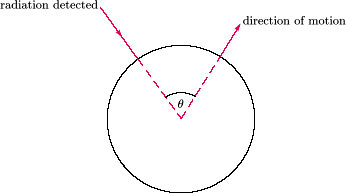Figure 26 The radiation detected at an angle θ to the direction of motion has a thermal spectrum characterised by a temperature T(v, θ) which depends on the speed v and the angle θ.

Not only does the Earth's motion affect the intensity as a function of angle, but also the energy of the photons. Suppose the Earth is moving with a velocitywith respect to the frame in which the radiation is isotropic and we observe the component of the 3 K radiation that arrives in a direction making an angle θ with our direction of motion (see Figure 26). What sort of spectrum would we observe? One might think that the observed spectrum would be very complicated. After all, the photons have been shifted to different frequencies, because of the Doppler effect, and our clocks and measuring rods will measure different intervals of time and length, compared with clocks and measuring rods in the ‘frame of isotropic 3 K radiation’. But it turns out that the result is very simple: Cosmic microwave radiation observed at an angle θ relative to the observer's direction of motion will still have a thermal spectrum, though it will be characterised by a temperature T(, θ) which depends on the observer's speedand the angle θ. (This reminds us of how the expansion of the Universe affected the cosmic background radiation: in that case too the thermal character of the spectrum was maintained; only the temperature was changed.)

It is in fact easy to calculate how T(, θ) depends onand θ. We shall use the fact that the frequency fmax, corresponding to the maximum intensity, is proportional to the temperature (see Question 10). That means that in the ‘rest frame’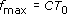where C is a constant and T0 (≈ 3 K) is the temperature of the radiation in the frame in which it is isotropic. But when we are moving with a speedand observing at an angle θ, we find a different frequency, fmax, corresponding to maximum intensity: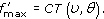The relationship between fmax and fmax is given by the formula for the relativistic Doppler shift: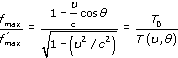Thus we find that

Thus, if we were to measure the apparent temperature of the radiation at different angles, we could determine our speedand the direction in which we are moving (which is the direction in which the radiation has the highest temperature, corresponding to θ = 0).

Radio astronomers measure the intensity of radiation at a particular frequency or over a particular range of frequencies. The variation of intensity with angle is particularly simple if measurements are taken at low frequencies, well below the frequency for maximum intensity, fmax. In this region of the spectrum, the intensity at a given frequency is proportional to the temperature (see Equation 8),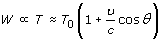and hence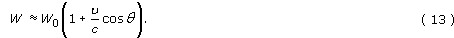The prediction of Equation 13 is plotted in Figure 27 for values of/c = 0, 0.1 and 0.2. The angular distributions (b) and (c) show a dipolar anisotropy.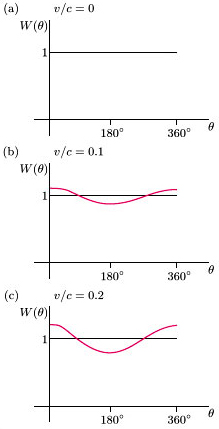Figure 27 The radiation intensity at low frequencies, W(θ), as a function of θ, for v/c equal to (a) 0, (b) 0.1 and (c) 0.2, where W(θ) is calculated from Equation 13. (Note that v/c for the Earth's motion round the Sun is only 10−4)

At higher frequencies, the coefficient of cos θ is more complicated and must be calculated from Equation 7. The result of such a calculation, which applies at all frequencies, is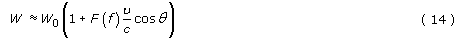where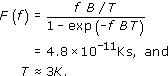But for fT/B ≈ 6 × 1010 Hz, we have F(f) ≈ 1 and obtain the simpler result of Equation 13.